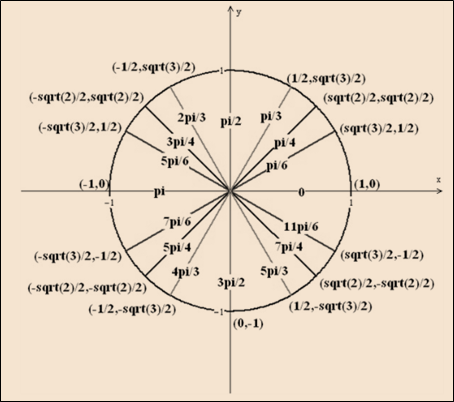# The value of x to satisfy the following inequality equation: sin x ≤ 1 2### Single Variable Calculus: Concepts...

4th Edition
James Stewart
Publisher: Cengage Learning
ISBN: 9781337687805### Single Variable Calculus: Concepts...

4th Edition
James Stewart
Publisher: Cengage Learning
ISBN: 9781337687805

#### Solutions

Chapter C, Problem 33E
To determine

## To calculate: The value of x to satisfy the following inequality equation:   sinx≤12

Expert Solution

The values of x for the interval [0,2π] to satisfy eq. sinx12 are [0xπ6] and [5π6xπ] .

### Explanation of Solution

Given information:

sinx12[0,2π]

Calculation:

Consider

The unit circle with co-ordinates (x,y)=(cosx,sinx) and radius 1 to find out the values of x for the interval [0,2π] :Fig. Unit circle

From the unit circle figure:

The y coordinate or sine value is equal to 12 at x=π6 and x=5π6 .

Sine value is less than or equal to 12 in the first quadrant for the x value of interval [0xπ6] . Sine is between 0 and 12 , for the x value of interval [5π6xπ] .

As in the entire fourth and third quadrant, the y value is negative so, sine should be negative and it is less than 12 for interval [πx2π] .

Therefore, the values of x for the interval [0,2π] to satisfy eq. sinx12 are [0xπ6] and [5π6xπ] .

### Have a homework question?

Subscribe to bartleby learn! Ask subject matter experts 30 homework questions each month. Plus, you’ll have access to millions of step-by-step textbook answers!